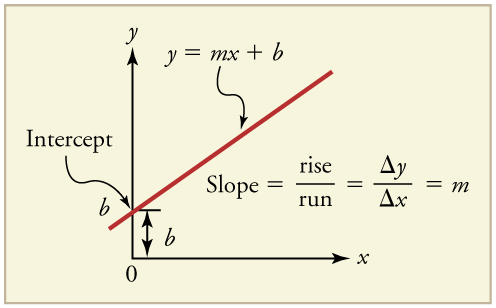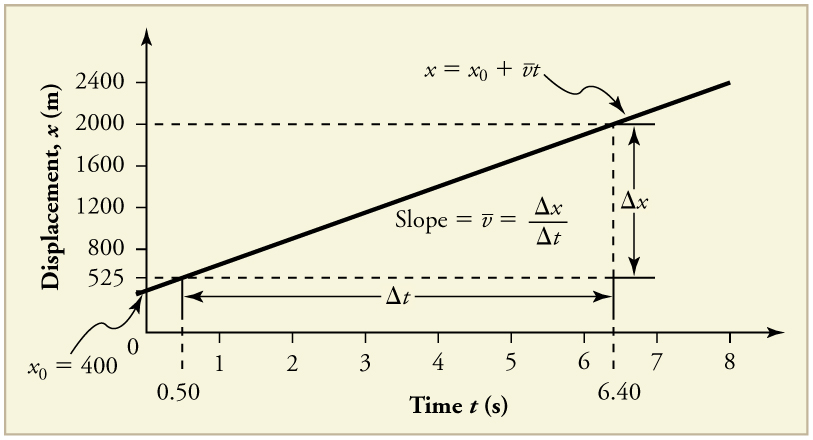# 2.8 Graphical analysis of one dimensional motion

 Page 1 / 8

## Learning objectives

By the end of this section, you will be able to:

• Describe a straight-line graph in terms of its slope and y -intercept.
• Determine average velocity or instantaneous velocity from a graph of position vs. time.
• Determine average or instantaneous acceleration from a graph of velocity vs. time.
• Derive a graph of velocity vs. time from a graph of position vs. time.
• Derive a graph of acceleration vs. time from a graph of velocity vs. time.

A graph, like a picture, is worth a thousand words. Graphs not only contain numerical information; they also reveal relationships between physical quantities. This section uses graphs of displacement, velocity, and acceleration versus time to illustrate one-dimensional kinematics.

## Slopes and general relationships

First note that graphs in this text have perpendicular axes, one horizontal and the other vertical. When two physical quantities are plotted against one another in such a graph, the horizontal axis is usually considered to be an independent variable    and the vertical axis a dependent variable    . If we call the horizontal axis the $x$ -axis and the vertical axis the $y$ -axis, as in [link] , a straight-line graph has the general form

$y=\text{mx}+b.$

Here $m$ is the slope    , defined to be the rise divided by the run (as seen in the figure) of the straight line. The letter $b$ is used for the y -intercept , which is the point at which the line crosses the vertical axis.A straight-line graph. The equation for a straight line is y = mx + b size 12{y= ital "mx"+b} {} .

## Graph of displacement vs. time ( a = 0, so v Is constant)

Time is usually an independent variable that other quantities, such as displacement, depend upon. A graph of displacement versus time would, thus, have $x$ on the vertical axis and $t$ on the horizontal axis. [link] is just such a straight-line graph. It shows a graph of displacement versus time for a jet-powered car on a very flat dry lake bed in Nevada.Graph of displacement versus time for a jet-powered car on the Bonneville Salt Flats.

Using the relationship between dependent and independent variables, we see that the slope in the graph above is average velocity $\stackrel{-}{v}$ and the intercept is displacement at time zero—that is, ${x}_{0}$ . Substituting these symbols into $y=\text{mx}+b$ gives

$x=\stackrel{-}{v}t+{x}_{0}$

or

$x={x}_{0}+\stackrel{-}{v}t.$

Thus a graph of displacement versus time gives a general relationship among displacement, velocity, and time, as well as giving detailed numerical information about a specific situation.

## The slope of x Vs. t

The slope of the graph of displacement $x$ vs. time $t$ is velocity $v$ .

$\text{slope}=\frac{\Delta x}{\Delta t}=v$

Notice that this equation is the same as that derived algebraically from other motion equations in Motion Equations for Constant Acceleration in One Dimension .

From the figure we can see that the car has a displacement of 400 m at time 0.650 m at $t$ = 1.0 s, and so on. Its displacement at times other than those listed in the table can be read from the graph; furthermore, information about its velocity and acceleration can also be obtained from the graph.

## Determining average velocity from a graph of displacement versus time: jet car

Find the average velocity of the car whose position is graphed in [link] .

Strategy

The slope of a graph of $x$ vs. $t$ is average velocity, since slope equals rise over run. In this case, rise = change in displacement and run = change in time, so that

$\text{slope}=\frac{\Delta x}{\Delta t}=\stackrel{-}{v}.$

Since the slope is constant here, any two points on the graph can be used to find the slope. (Generally speaking, it is most accurate to use two widely separated points on the straight line. This is because any error in reading data from the graph is proportionally smaller if the interval is larger.)

Solution

1. Choose two points on the line. In this case, we choose the points labeled on the graph: (6.4 s, 2000 m) and (0.50 s, 525 m). (Note, however, that you could choose any two points.)

2. Substitute the $x$ and $t$ values of the chosen points into the equation. Remember in calculating change $\left(\Delta \right)$ we always use final value minus initial value.

$\stackrel{-}{v}=\frac{\Delta x}{\Delta t}=\frac{\text{2000 m}-\text{525 m}}{6\text{.}\text{4 s}-0\text{.}\text{50 s}},$

yielding

$\stackrel{-}{v}=\text{250 m/s}.$

Discussion

This is an impressively large land speed (900 km/h, or about 560 mi/h): much greater than the typical highway speed limit of 60 mi/h (27 m/s or 96 km/h), but considerably shy of the record of 343 m/s (1234 km/h or 766 mi/h) set in 1997.

#### Questions & Answers

how vapour pressure of a liquid lost through convection
Yomzi Reply
Roofs are sometimes pushed off vertically during a tropical cyclone, and buildings sometimes explode outward when hit by a tornado. Use Bernoulli’s principle to explain these phenomena.
Aliraza Reply
Plz answer the question ☝️☝️
Aliraza
what's the basic si unit of acceleration
ELLOIN Reply
Explain why the change in velocity is different in the two frames, whereas the change in kinetic energy is the same in both.
Fabian Reply
Insulators (nonmetals) have a higher BE than metals, and it is more difficult for photons to eject electrons from insulators. Discuss how this relates to the free charges in metals that make them good conductors.
Muhammad Reply
Is the photoelectric effect a direct consequence of the wave character of EM radiation or of the particle character of EM radiation? Explain briefly.
Muhammad
Determine the total force and the absolute pressure on the bottom of a swimming pool 28.0m by 8.5m whose uniform depth is 1 .8m.
Henny Reply
how solve this problem?
Foday
P(pressure)=density ×depth×acceleration due to gravity Force =P×Area(28.0x8.5)
Fomukom
for the answer to complete, the units need specified why
muqaddas Reply
That's just how the AP grades. Otherwise, you could be talking about m/s when the answer requires m/s^2. They need to know what you are referring to.
Kyle
Suppose a speck of dust in an electrostatic precipitator has 1.0000×1012 protons in it and has a net charge of –5.00 nC (a very large charge for a small speck). How many electrons does it have?
Alexia Reply
how would I work this problem
Alexia
how can you have not an integer number of protons? If, on the other hand it supposed to be 1e12, then 1.6e-19C/proton • 1e12 protons=1.6e-7 C is the charge of the protons in the speck, so the difference between this and 5e-9C is made up by electrons
Igor
what is angular velocity
Obaapa Reply
angular velocity can be defined as the rate of change in radian over seconds.
Fidelis
Why does earth exert only a tiny downward pull?
Mya Reply
hello
Islam
Why is light bright?
Abraham Reply
what is radioactive element
Attah Reply
an 8.0 capacitor is connected by to the terminals of 60Hz whoes rms voltage is 150v. a.find the capacity reactance and rms to the circuit
Aisha Reply
thanks so much. i undersooth well
Valdes Reply
what is physics
Nwafor Reply
is the study of matter in relation to energy
Kintu
physics can be defined as the natural science that deals with the study of motion through space,time along with its related concepts which are energy and force
Fidelis

### Read also:

#### Get Jobilize Job Search Mobile App in your pocket Now!

Source:  OpenStax, College physics for ap® courses. OpenStax CNX. Nov 04, 2016 Download for free at https://legacy.cnx.org/content/col11844/1.14
Google Play and the Google Play logo are trademarks of Google Inc.

Notification Switch

Would you like to follow the 'College physics for ap® courses' conversation and receive update notifications?ByByBy Stephen VoronBy OpenStaxBy Jonathan LongByBy OpenStaxBy Monty HartfieldBy Tod McGrathBy JavaChamp TeamBy OpenStaxBy OpenStax